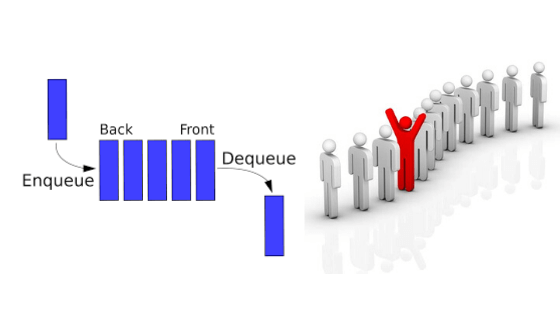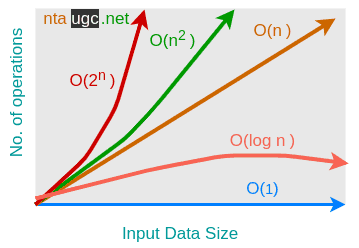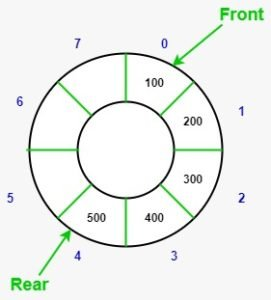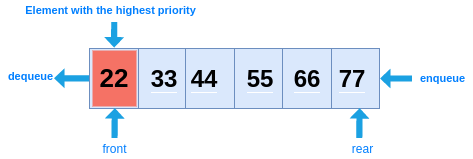# Queue## Types Of Queue In Data Structure (Queue Variations)

The standard queue data structures have the following variations:

• Simple Queue (Queue)
• Double-ended Queue (deque)
• Circular Queue
• Priority Queue

## What Is The Queue And How It Differs From The Stack

• We can also say that a queue is a sequentially ordered list where insertions are done from one end (rear) and deletions are done from another end (front)
• A Queue follow the first-in-first-out (FIFO) principle.
• Elements may be inserted at any time, but only the element which has been in the queue the longest maybe removal.
• In Enqueue ( )  operation only rear pointer move rather than front and in Dequeue ( ) operation only front pointer moves.
• If front = rear = -1 i.e; Queue is empty or running underflow condition.
• If front = rear than only one element in the queue.
• Index of the front is greater than the rear than the queue is empty.
• If rear = n-1 (index) where the n = size of a queue than queue is full; this condition is also a big drawback of the linear queue for this solution we used circular queue which we briefly discuss in circular queue variations.
• First person on a queue is called “front” and last is known as “rear”
• Queue dequeue operation and queue enqueue operation similar as stack pop and push operation
• The time complexity of queue operation is O(1)Elements are always added from the back and removed from the front. Think of it as a line of people waiting for a railway ticket. The person who is at the beginning of the line will get the first ticket.

## The Queue Supports The Following Fundamental Methods

• New() : ADT – Creates and empty queue
• Enqueue ( S : ADT, O : element) : ADT – inserts object O at the rear of the queue
• Dequeue ( S : ADT ) : ADT – Removes the object from the front of the queue, and error occurs if the queue is empty.
• Front ( S : ADT ) : element – Returns, but does not remove, the front element, and error occurs if the queue is empty

• queue[]
• arraySize
• front
• rear

## Axioms

• Front ( Enqueue ( New (), V )) = V
• Dequeque ( Enqueue ( New (), V ))
• Front ( Enqueue (Enqueue ( Q, W ), V )) = Front ( Enqueue ( Q, W ))
• Dequeue ( Enqueue ( Enqueue ( Q, W ) , V )) = Enqueue ( Dequeue ( Enqueue ( Q, W )), V )

• Printer
• Redo / Undo
• Highway Toll

## Deque (Double-ended Queue)Deque is pronounced deck.

• Deque supports both stack and queue operations
• Insertion and deletion are allowed at both ends of the queue.

• So we can say a queue where to enqueue and dequeue is allowed both the end. It is a generalized version of a linear queue  ( Queue )

## Operations on Deque:

The Deque Supports The Following Fundamental Methods

insertFront()
insertLast()
deleteFront()
deleteLast()

similarly to the above operations, the following operations are also supported
getFront()
getRear()
isEmpty()
isFull()

## Types of Deque

### Input Restricted Deque

As the name says, insertion (enqueue) can be only one end but deletion (dequeue) is possible from both end (rear and, front).

### Output restricted Deque

In output restricted, Deletion (dequeue) can be possible from one end and insertion (enqueue) can be both ends.

## Circular Queue

It is also well known as ‘Ring Buffer’.circular Queue is a linear data structure wherein the operations are executed based on the concept of FIFO (First In First Out) principle and the last position is attached back to the first position to make a circle.

We can insert elements in a simple Queue until the queue gets full. But once the queue is complete, even if there is a space in front of the queue we can not insert the next items.

## Operations on Circular Queue:

• Front: Get the first item
• Rear: Get the Nth item where Nth refers to last element
• deQueue()  For circular queue, deQueue() remove element from front or first position

## Priority QueueA priority queue is a more specific or expanding data structure of a simple queue. The major difference properties are as follows.

1. Priority associated with every elements.
2. A high priority item is dequeued first.
3. when two elements are given the same priority than dequeued according to the queue order.

## Basic Operations

• insert / enqueue

•  remove / dequeue

• Peek

•  isFull

• isEmpty

## Priority Queue Application

Application of priority queue is as follow : –

• Stack implementation.
• Interrupt handling and load balancing in an operating system.
• In Huffman code for data compression.
• Dijkstra’s algorithm.